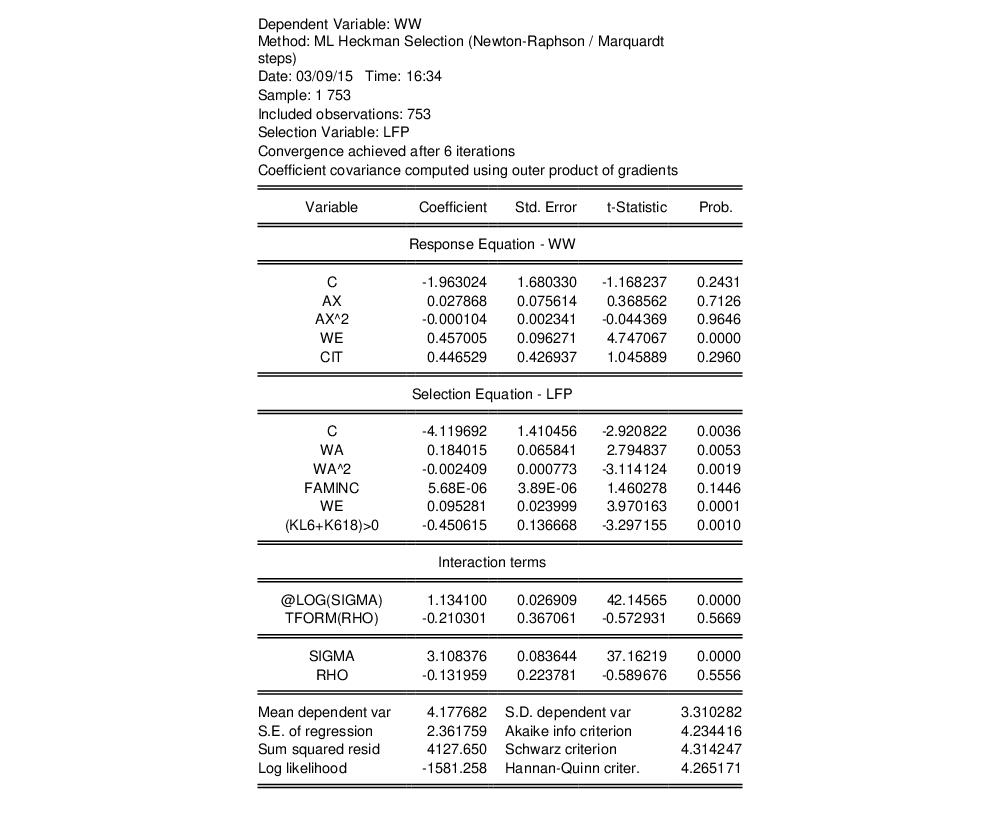User’s Guide : Advanced Single Equation Analysis : Discrete and Limited Dependent Variable Models : Heckman Selection Model

Heckman Selection Model
The Heckman (1976) selection model, sometimes called the Heckit model, is a method for estimating regression models which suffer from sample selection bias. Under the Heckman selection framework, the dependent variable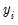is only observable for a portion of the data. A classic example, in economics, of the sample selection problem is the wage equation for women, whereby a woman’s wage is only observed if she makes the decision to enter the work place, and is unobservable if she does not. Heckman’s (1976) paper that introduced the Heckman Selection model worked on this very problem.
EViews provides an estimator for the simple linear Heckman Selection Model. This model is specified as: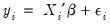(31.38)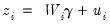(31.39)
where is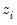a binary variable, withonly observed when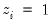.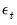and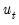are error terms which follow a bivariate normal distribution:(31.40)
with scale parameter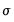and correlation coefficient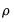. Note that we have normalized the variance ofto 1 since this variance is not identified in this model.
Equation Equation (31.38) is generally referred to as the response equation, withthe variable of interest. Equation Equation (31.39) is termed the selection equation and determines whether isobserved or not.
EViews offers two different methods of estimating this model: Heckman’s original two-step method and a Maximum Likelihood method.
The Heckman Two-Step Method
The Heckman two-step method is based around the observation that: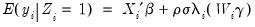(31.41)
where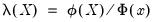is the Inverse Mills Ratio (Greene, 2008), and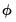and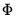are the standard normal density and cumulative distribution function, respectively. Then we may specify a regression model: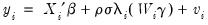(31.42)
The two-step method proceeds by first estimating a Probit regression for Equation (31.39) to obtain an estimate of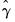, from which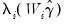may be calculated. A least squares regression ofon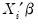and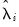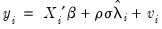(31.43)
is then computed, yielding consistent estimates of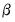and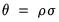. An estimator for the error standard deviationmay be obtained from the usual standard error of the regression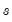, and the ratio estimator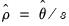follows.
The estimator of the coefficient covariance matrix of the two-step method is given by: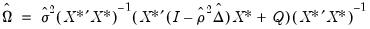(31.44)
where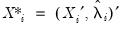,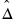is a diagonal matrix with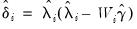on the diagonals,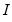is an identity matrix,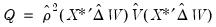, and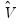is the coefficient covariance matrix from the Probit estimation of Equation (31.39).
Maximum Likelihood
The maximum likelihood method of estimating the Heckman Selection Model is performed using the log-likelihood function given by: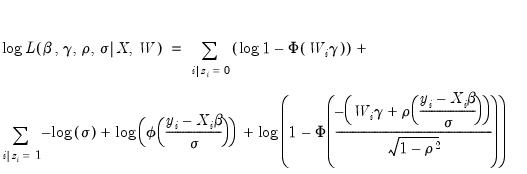(31.45)
where the first summation is over observations for which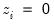(i.e., whenis unobserved), and the second for observations for which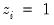(i.e., whenis observed).
It is straightforward to maximize this log-likelihood function with respect to the parameters,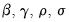. However, this maximization is unrestricted with regards toand, when, in fact, there are restrictions of the form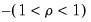and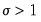imposed on the parameters. EViews optimizes the model using transformed versions of the parameters: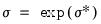(31.46)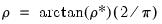(31.47)
to impose the restrictions.
Starting values for the optimization can be obtained using the Heckman two-step method outlined above.
As with most maximum likelihood estimations, the covariance matrix of the estimated parameters can be calculated as either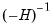(where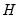is the Hessian matrix, the information matrix),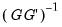(where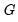is the matrix of gradients), or as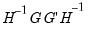(the Huber/White matrix).
Estimating the Heckman Selection Model in EViews
To estimate the Heckman Selection Model, open the equation dialog by selecting Quick/Estimate Equation… or Object/New Object…/Equation in the main EViews menu and selecting Heckit from the Method dropdown menu. Alternately, you may enter the command heckit in the command line.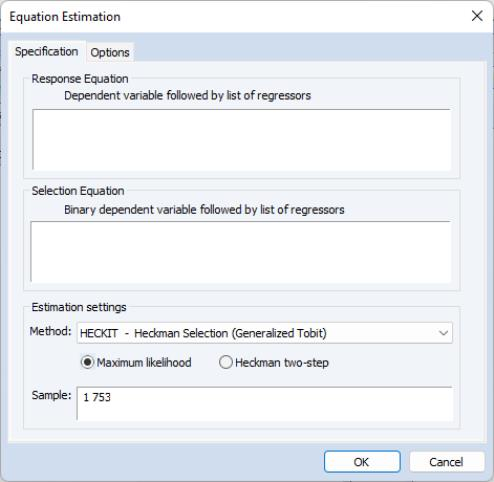The first page of the dialog, the Specification tab, lets you specify both the response equation Equation (31.38) and the selection equation Equation (31.39). Both equations should be specified as the dependent variable followed by a space delimited list of regressors. Note that the dependent variable for the selection equation should be series containing only zeros and ones.
The Specification page also lets you select the type of estimation method by selecting one of the two radio buttons; either Maximum Likelihood or Heckman two-step.
If you have chosen to estimate via maximum likelihood, the Options tab of the dialog lets you specify the type of covariance matrix, by using the Coefficient covariance matrix dropdown menu. You may choose from Outer Product of Gradients, Information Matrix, and Huber/White. You may also choose starting values for the maximum likelihood procedure. You may select EViews Supplied to perform the Heckman two-step procedure to obtain starting values, or you can down-weight those starting values by choosing a multiple of them. The User Supplied option will use the specified coefficient vector in the workfile to obtain starting values.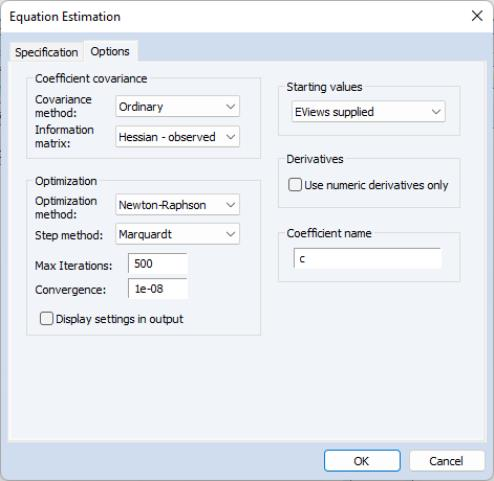An Example
As an example of the estimation of the Heckman Selection model, we take one of the results from Econometric Analysis by William H. Greene (6th Edition, p. 888, Example 24.8), which uses data from the Mroz (1987) study of the labor supply of married women to estimate a wage equation for women. Only 428 of the 753 women studied participated in the labor force, so a selection equation is provided to model the sample selection behavior of married women.
The wage equation is given by: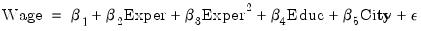(31.48)
where EXPER is a measure of each woman’s experience, EDUC is her level of education, and CITY is a dummy variable for whether she lives in a city or not.
The selection equation is given by: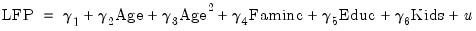(31.49)
where LFP is a binary variable taking a value of 1 if the woman is in the labor force, and 0 otherwise, AGE is her age, FAMINC is the level of household income not earned by the woman, and KIDS is a dummy variable for whether she has children.
You can bring the Mroz data directly into EViews from Greene’s website, using the following EViews command:
wfopen http://www.stern.nyu.edu/~wgreene/Text/Edition7/TableF5-1.txt
In this data, the wage data are in the series WW, experience is AX, education is in WE, the city dummy is CIT, labor force participation is LFP, age is WA, and family income is FAMINC. There is no kids dummy variable, but there are two variables containing the number of children below K6 education (KL6), and the number of kids between K6 education and 18 (K618). We can create the dummy variable simply by testing whether the sum of those two variables is greater than 0.
To estimate this equation in EViews, we click on Quick/Estimate Equation…, and then change the equation method to Heckit. In the Response Equation box we type:
ww c ax ax^2 we cit
And in the Selection Equation box we type:
lfp c wa wa^2 faminc we (kl6+k618)>0
To begin we select the Heckman two-step estimation method. After clicking OK, the estimation results show and replicate the results in the first pane of Table 24.3 in Greene (note that Greene only shows the estimates of the Wage equation, plusand).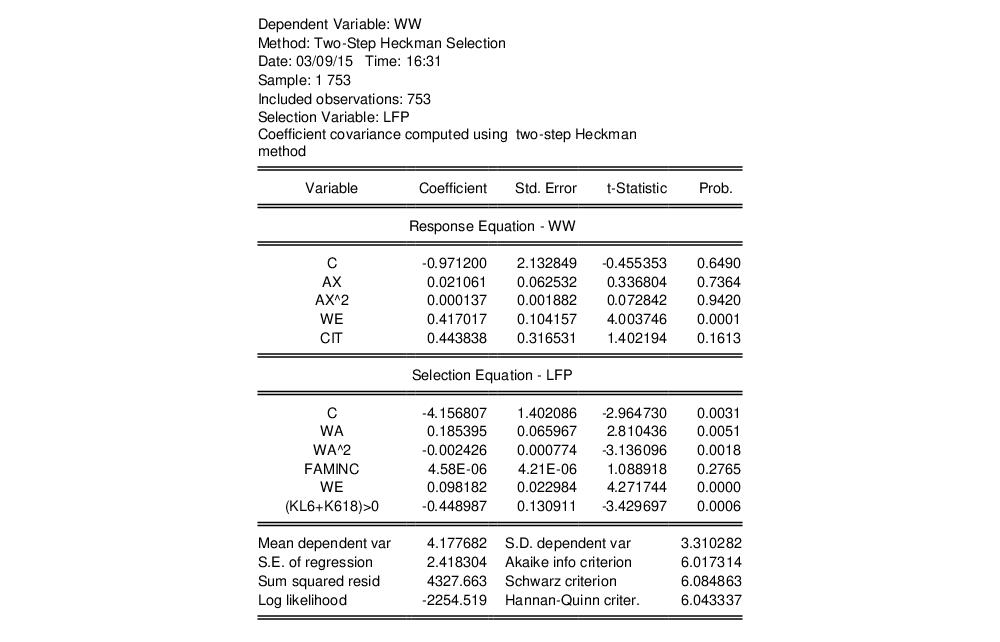We can modify our equation to use as the estimation method. Click on the Estimate button to bring up the estimation dialog and change the method to Maximum Likelihood. Next, click on the Options tab and change the Information matrix to OPG and click on OK to estimate the equation. The results match the second pane of Table 24.3 in Greene.